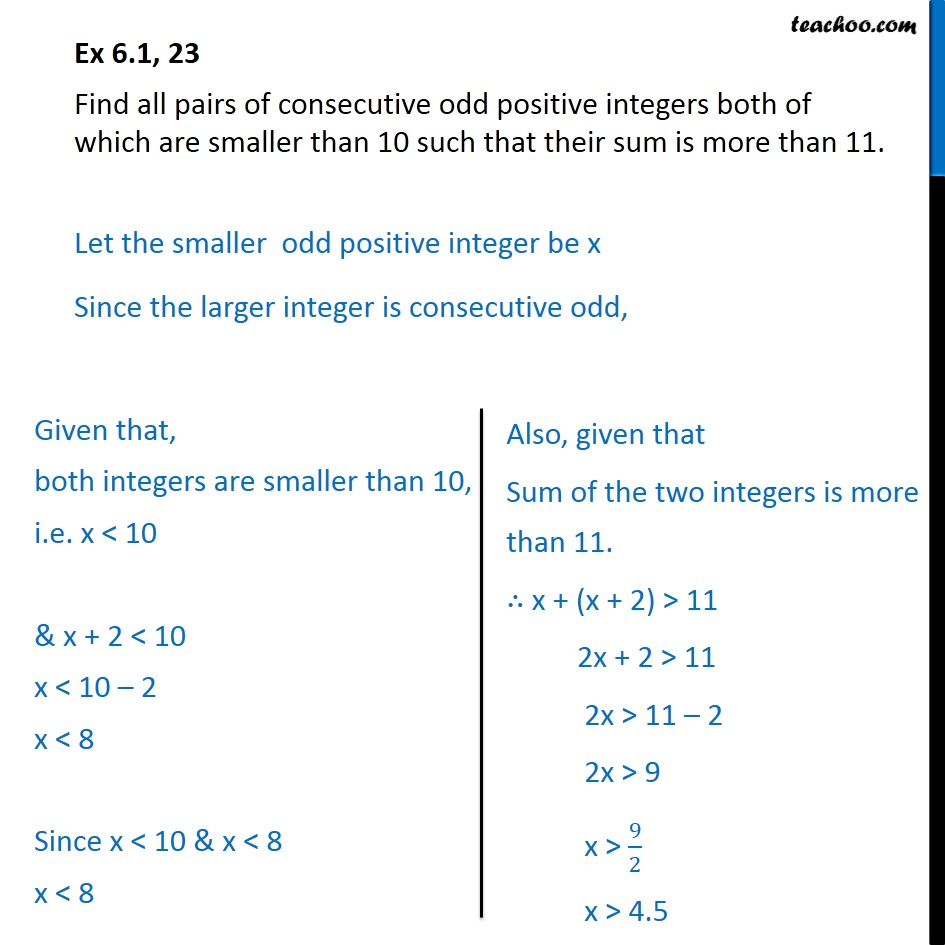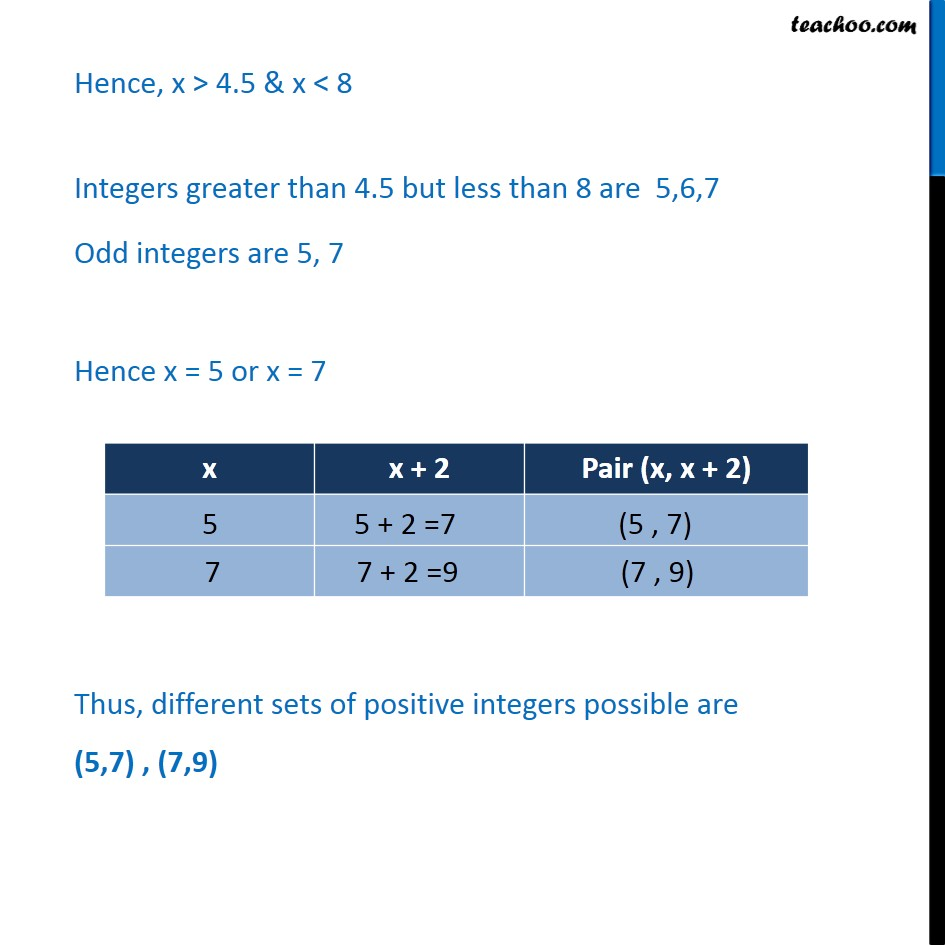1. Class 11
2. Important Question for exams Class 11
3. Chapter 6 Class 11 Linear Inequalities

Transcript

Ex 6.1, 23 Find all pairs of consecutive odd positive integers both of which are smaller than 10 such that their sum is more than 11. Let the smaller odd positive integer be x Since the larger integer is consecutive odd, Hence, x > 4.5 & x < 8 Integers greater than 4.5 but less than 8 are 5,6,7 Odd integers are 5, 7 Hence x = 5 or x = 7

Chapter 6 Class 11 Linear Inequalities

Class 11
Important Question for exams Class 11